﻿ 鱿鱼内脏活性肽的提取工艺优化及功能评价 Extraction Optimization and Function Evaluation of Peptides from Squid Viscera

Hans Journal of Food and Nutrition Science
Vol.07 No.03(2018), Article ID:26277,12 pages
10.12677/HJFNS.2018.73020

Extraction Optimization and Function Evaluation of Peptides from Squid Viscera

Jiamin Zhou, Tingting Li*, Yuanheng Wang, Xuan He

Key Laboratory of Biotechnology and Bioresources Utilization (Dalian Minzu University), Ministry of Education, Dalian Liaoning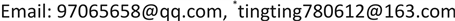Received: Jul. 19th, 2018; accepted: Jul. 30th, 2018; published: Aug. 6th, 2018ABSTRACT

In this study, the squid viscera was used as the main raw material, and the effect of trypsin on the degree of hydrolysis and the antioxidant activity of the hydrolysate were investigated. The effects of hydrolysis temperature, time, pH, and amount of enzyme on the degree of hydrolysis were investigated using single-factor and orthogonal experiments. Response surface methodology was used to optimize the hydrolysis conditions. The results showed that the optimal conditions for enzymatic hydrolysis of squid viscera were pH 7.04, enzymolysis temperature 42.43˚C, enzymolysis time 6.98 h, enzyme addition amount 5250 U/g, and the degree of hydrolysis after enzymatic hydrolysis of squid viscera was 82.906%. To verify the feasibility of the response surface model, the modified optimal conditions were used: IE pH 7.0, enzymatic hydrolysis temperature 43˚C, enzymolysis time 7.0 h, enzymatic addition of 5250 U/g for enzymatic digestion test of squid viscera, and the actual average of the sub-parallel test was 81.836%, and the relative deviation from the prediction value obtained by the regression equation was 1.31%. And hydroxyl radical scavenging rate was 28.9% and the degree of hydrolysis was 74.25% using the optimal conditions of enzymatic hydrolysis. The results showed that the squid viscera had a certain degree of antioxidant activity.

Keywords:Squid Viscera, Degree of Hydrolysis, Hydroxyl Radical Scavenging Rate, Response Surface MethodologyCopyright © 2018 by authors and Hans Publishers Inc.1. 引言

2. 材料与方法

2.1. 材料与仪器

2.2. 实验方法

2.2.1. 单因素实验设计

2.2.2. 酶解

2.2.3. 总氨基氮含量的测定

$X=\frac{\left({V}_{1}-{V}_{2}\right)×C×\frac{14}{1000}}{5×\frac{{V}_{3}}{100}}×1000×100$ (1)

2.2.4. 总氮的含量测定

$X=\frac{\left({V}_{1}-{V}_{2}\right)×C×0.014}{M×\frac{10}{100}}×100$ (2)

2.2.5. 水解度的计算

2.2.6. 羟自由基清除率的计算

$D%=\frac{\left({A}_{测定}-{A}_{标准}\right)}{\left({A}_{空白}-{A}_{标准}\right)}×100%$ (3)

3. 结果与分析

3.1. 单因素实验

3.1.1. PH对胰蛋白酶水解度的影响

3.1.2. 加酶量对胰蛋白酶水解度的影响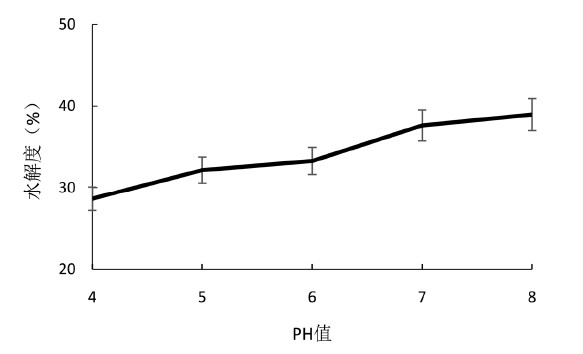Figure 1. The influence of pH on the hydrolysis degree of trypsin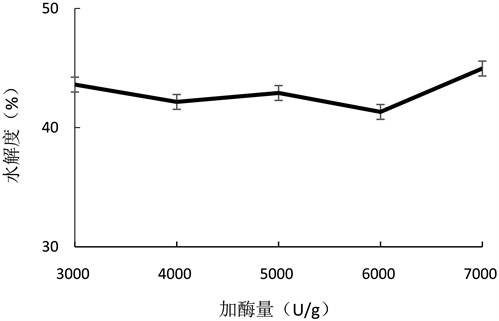Figure 2. The influence of enzyme concentration on the hydrolysis degrees of trypsin

3.1.3. 温度对胰蛋白酶水解度的影响

3.1.4. 时间对胰蛋白酶水解度的影响

3.2. 酶解条件的优化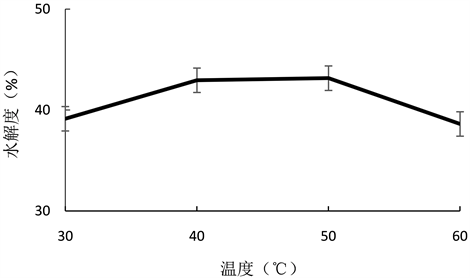Figure 3. The influence of temperature on the hydrolysis degrees of trypsin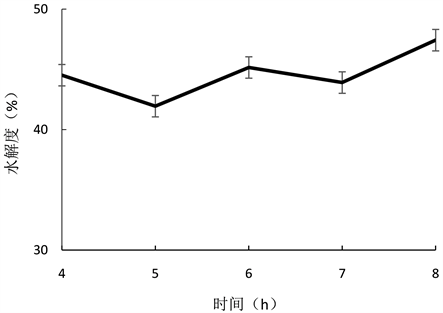Figure 4. The influence of time on the hydrolysis degrees of trypsin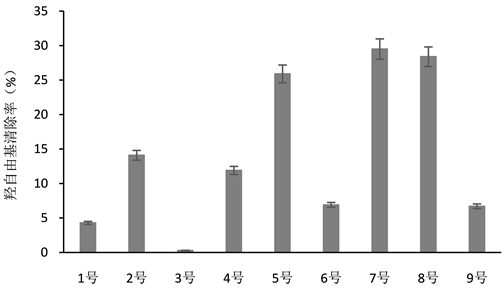Figure 5. Orthogonal hydroxyl radical scavenging rate of trypsinTable 1. Orthogonal experimental factors of trypsin

3.3. 响应面法优化鱿鱼内脏酶解工艺的研究

3.3.1. 实验设计及结果分析

$\begin{array}{c}水解度=\text{78}.\text{39}+\text{1}.0\text{3A}+\text{3}.\text{8}0\text{B}+\text{2}.\text{13C}+\text{15}.\text{63D}+0.\text{84AB}+\text{5}.\text{92AC}-\text{2}.\text{56AD}\\ +\text{3}.\text{51BC}+\text{6}.\text{64BD}+\text{3}.\text{57CD}-\text{14}.{\text{57A}}^{\text{2}}-\text{15}.{\text{93B}}^{\text{2}}-\text{13}.{\text{22C}}^{\text{2}}-\text{18}.{\text{14D}}^{\text{2}}\end{array}$

3.3.2. 各因素之间的交互作用Table 3. Experimental factors of the response surfaceTable 5. Variance analysis of the regression equationFigure 6. The influence of pH value and enzymolysis temperature on the hydrolysis degree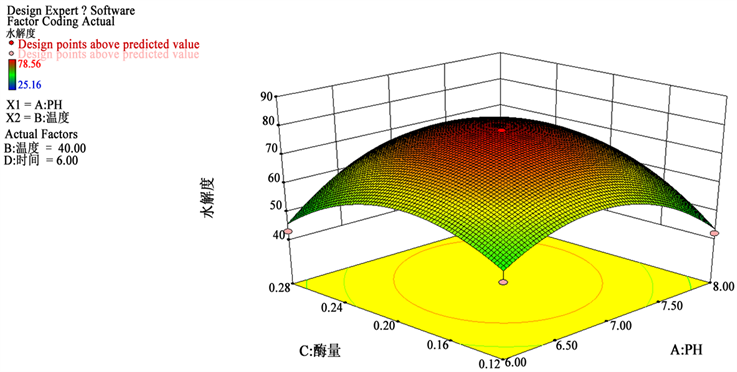Figure 7. The influence of pH value and enzyme concentration on the hydrolysis degree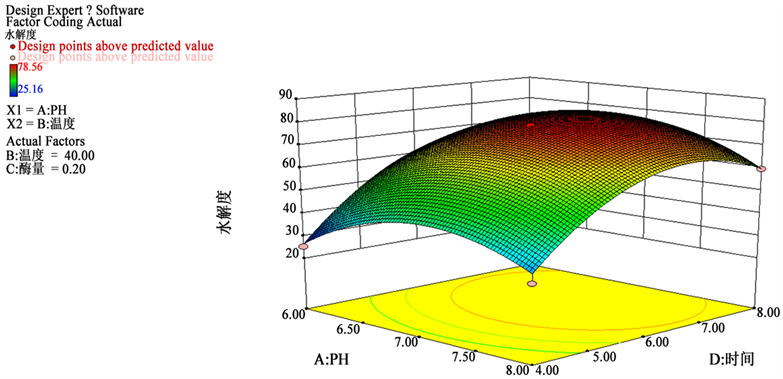Figure 8. The influence of pH value and enzymolysis time on the hydrolysis degree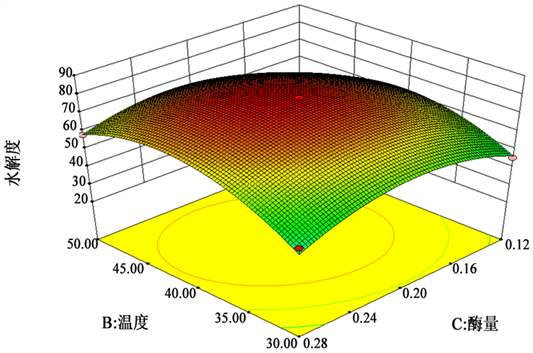Figure 9. The influence of temperature and concentration of enzymolysis on the hydrolysis degree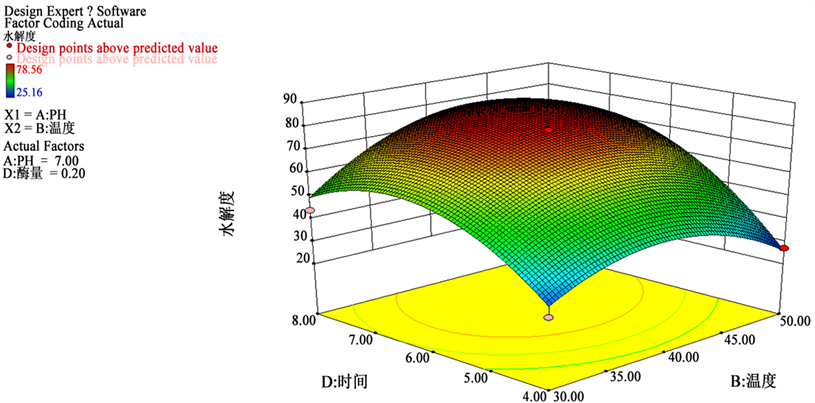Figure 10. The influence of time and temperature of enzymolysis on the hydrolysis degree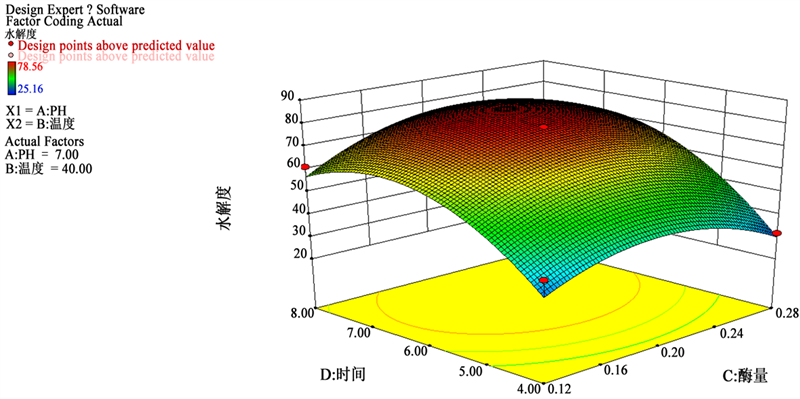Figure 11. The influence of time and concentration of enzymolysis on the hydrolysis degree

3.3.3. 酶解工艺条件的验证

4. 结论

Extraction Optimization and Function Evaluation of Peptides from Squid Viscera[J]. 食品与营养科学, 2018, 07(03): 166-177. https://doi.org/10.12677/HJFNS.2018.73020

1. 1. 朱瑶, 胡建恩, 杨杰, 等. 鱿鱼肝脏活性肽的制备及生物活性研究[J]. 安徽农业科学, 2014, 42(15): 4666-4668.

2. 2. GB/T 9725-2007, 化学试剂电位滴定法通则[S].

3. 3. GB/T 5009.5-2003, 食品中蛋白质的测定[S].

4. NOTES

*通讯作者。